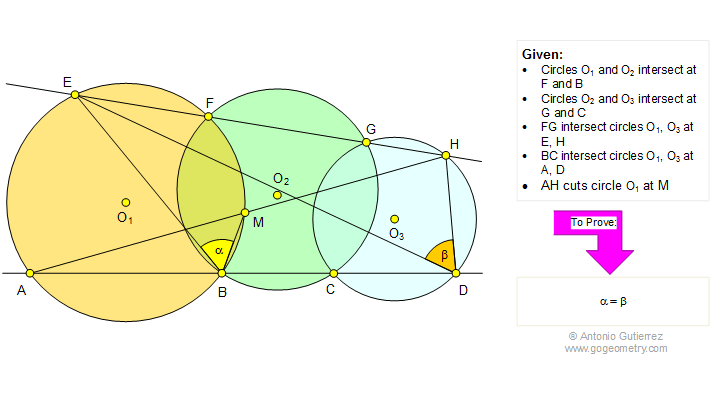Problem 74. Three Intersecting Circles, Cyclic Quadrilateral, Angles. Level: High School, SAT Prep, College

 In the figure below, the circles O1 and O2 intersect at F and B. The circles O2 and O3 intersect at G and C. FG intersect circles O1 and O3 at E and H, respectively. BC intersect circles O1 and O3 at A and D, respectively. AH cuts circle O1 at M. Prove that angles EBM and EDH are equal. View or post a solution.Home | Geometry | Search | Problems | 71-80 | Common Chord | Email